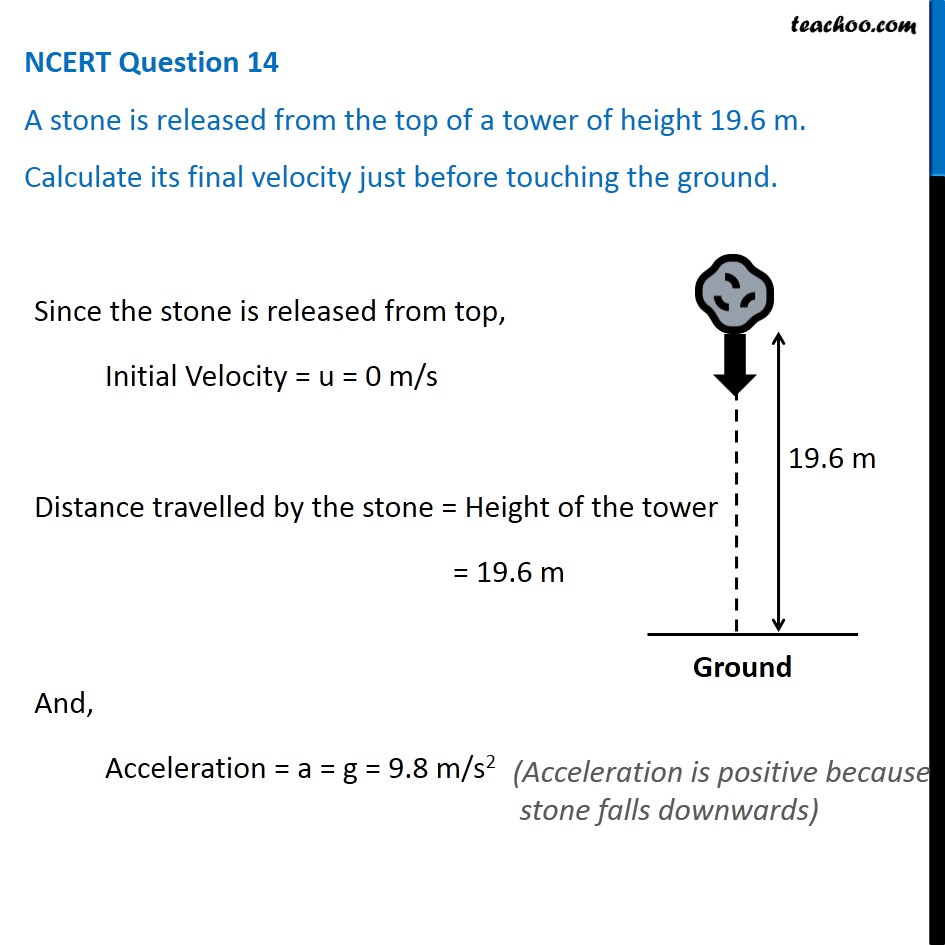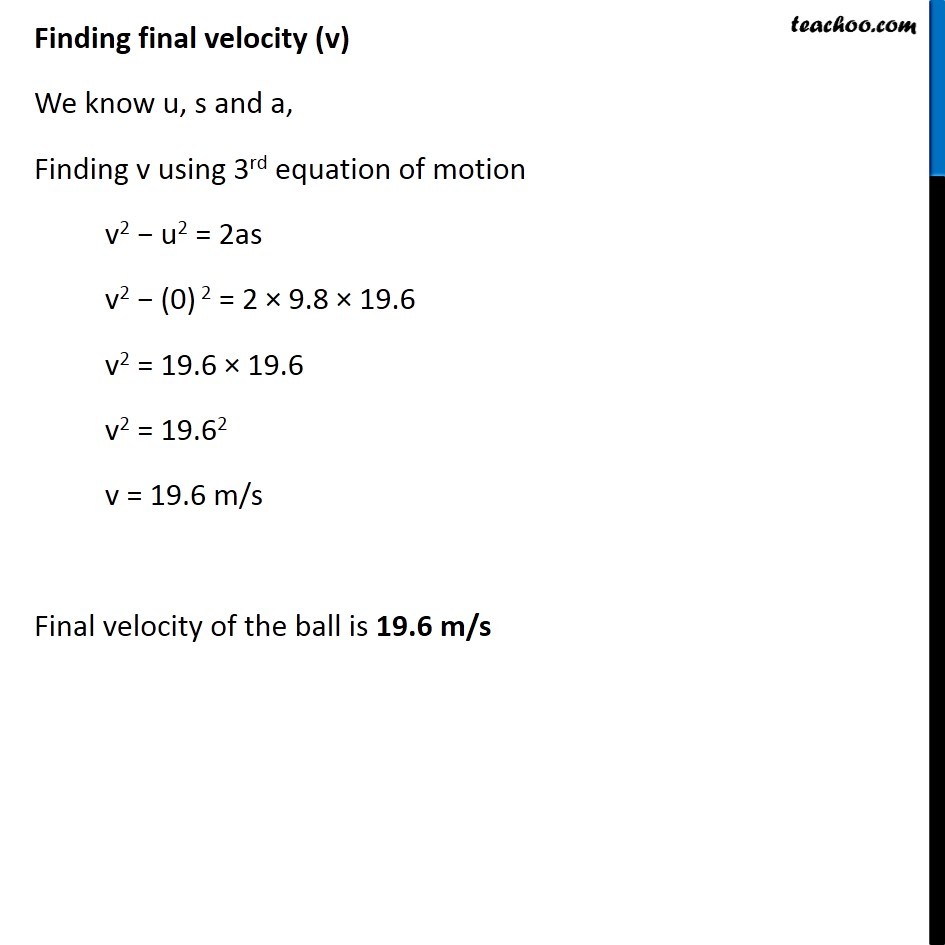NCERT Questions

Class 9
Chapter 10 Class 9 - GravitationLearn in your speed, with individual attention - Teachoo Maths 1-on-1 Class

### Transcript

NCERT Question 14 A stone is released from the top of a tower of height 19.6 m. Calculate its final velocity just before touching the ground. Since the stone is released from top, Initial Velocity = u = 0 m/s Distance travelled by the stone = Height of the tower = 19.6 m And, Acceleration = a = g = 9.8 m/s2 (Acceleration is positive because stone falls downwards) Finding final velocity (v) We know u, s and a, Finding v using 3rd equation of motion v2 − u2 = 2as v2 − (0) 2 = 2 × 9.8 × 19.6 v2 = 19.6 × 19.6 v2 = 19.62 v = 19.6 m/s Final velocity of the ball is 19.6 m/s